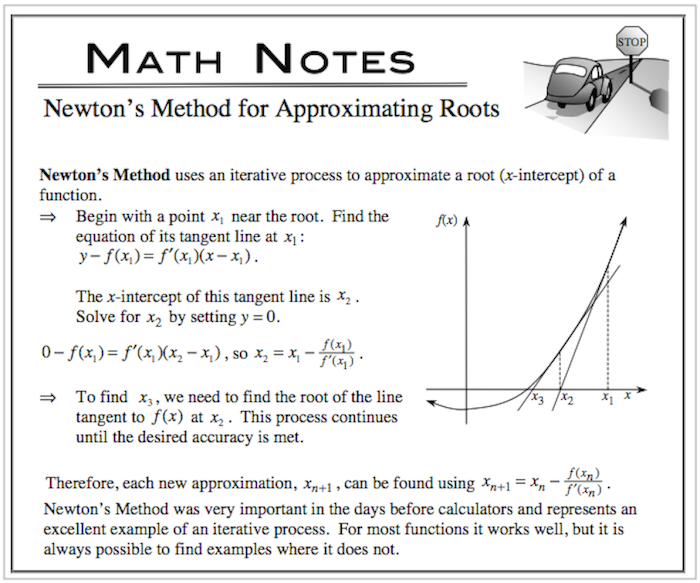### Home > CALC > Chapter 8 > Lesson 8.4.1 > Problem8-141

8-141.

Without looking at the graph, use Newton's Method for approximating roots for $f(x) =\frac { 1 } { 1 + x ^ { 2 } }$ if $x_1 = 1$. What happened?

What happened?
Think about the shape of the graph of $f(x)$.
What is its range? What does that mean about the roots?